# A steel rod, which is free to move, has a length of 200 mm and diameter of 20 mm at a temperature of 15°C. If the rod is heated uniformly to 115°C,determine the length and the diameter of this rod to the nearest micron at the new temperature if the linear coefficient of thermal expansion of steel is 12.5 * 10-6m/m/C. Is there a stress on the rod at 115°C?If the rod is snugly fitted against two immovable nonconducting walls at a temperature of 15°C and then heated uniformly to115°C, what is its length at 115°C? If the modulus of elasticity of steel is207 GPa, what is the stress induced in the bar? Is this stress tension or compression?

Question
1 views

A steel rod, which is free to move, has a length of 200 mm and diameter of 20 mm at a temperature of 15°C. If the rod is heated uniformly to 115°C,determine the length and the diameter of this rod to the nearest micron at the new temperature if the linear coefficient of thermal expansion of steel is 12.5 * 10-6m/m/C. Is there a stress on the rod at 115°C?If the rod is snugly fitted against two immovable nonconducting walls at a temperature of 15°C and then heated uniformly to115°C, what is its length at 115°C? If the modulus of elasticity of steel is207 GPa, what is the stress induced in the bar? Is this stress tension or compression?

check_circle

Step 1

Given:

The initial length of the rod is 200 mm.

The initial diameter of the rod is 20 mm.

The linear coefficient of thermal expansion of steel is 12.5 x 10-6m/m/C.

The modulus of elasticity of steel is 207 GPa.

Step 2

The formula to calculate the final length of the rod is,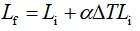The formula used to calculate the final diameter of the rod is,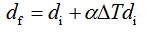The formula used to calculate the stress is,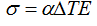Step 3

Calculate the gross volume of the final length of the rod.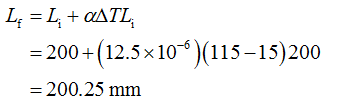Calculate the final diameter of the rod.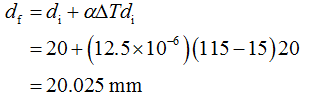Since the rod is free to move so, there will be no stress on the rod at 115°C.

...

### Want to see the full answer?

See Solution

#### Want to see this answer and more?

Solutions are written by subject experts who are available 24/7. Questions are typically answered within 1 hour.*

See Solution
*Response times may vary by subject and question.
Tagged in